Next: Flow over Shallow Bump Up: Incompressible Inviscid Flow Previous: Flow Through an Orifice

# Sub-Critical and Super-Critical Flow

Consider a shallow stream of depth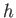, uniform width, and uniform flow velocity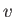, that is fed from a deep reservoir whose surface lies a heightabove the (horizontal) bed of the stream. Here,is usually referred to as the head height. Assuming that the water in the reservoir is effectively stationary, application of Bernoulli's equation to a streamline lying on the surface of the water (where the pressure is atmospheric) yields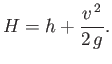(4.18)

Letbe the flow rate per unit width of the stream, which is assumed to be fixed. It follows that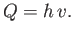(4.19)

The previous two equations can be combined to give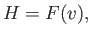(4.20)

where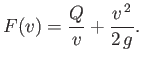(4.21)

It is easily demonstrated that the function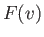attains its minimum value,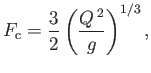(4.22)

when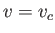, where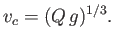(4.23)

We conclude that, as long as, Equation (4.20) possesses two possible solutions that are consistent with a given head height and flow rate. In one solution, the stream flows at a relatively slow velocity,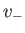, which is such that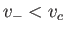. In the other, the stream flows at a relatively fast velocity,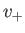, which is such that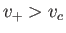. The corresponding depths areand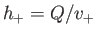, respectively.

It is helpful to introduce the dimensionless Froude number,(4.24)

(See Section 1.15.) Note thatis the characteristic propagation velocity of a gravity wave in shallow water of depth. (See Section 11.4.) Hence, ifthen the stream's flow velocity falls below the wave speed--such flow is termed sub-critical. On the other hand, if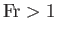then the flow velocity exceeds the wave speed--such flow is termed super-critical.

We can combine Equations (4.18), (4.19), and (4.24) to give(4.25)

where(4.26)

It is easily demonstrated thatattains its minimum value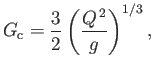(4.27)

when, where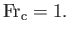(4.28)

Hence, we again conclude that, as long as, there are two possible flow velocities of the stream (parameterized by two different Froude numbers) that are consistent with a given head height and flow rate. However, it is now clear that the smaller velocity is sub-critical (i.e.,), whereas the larger velocity is super-critical (i.e.,).Next: Flow over Shallow Bump Up: Incompressible Inviscid Flow Previous: Flow Through an Orifice
Richard Fitzpatrick 2016-03-31